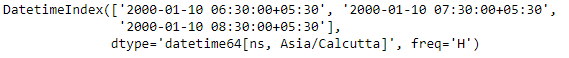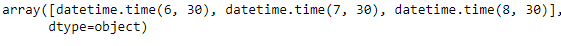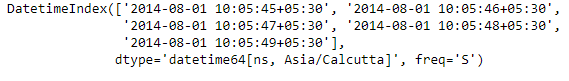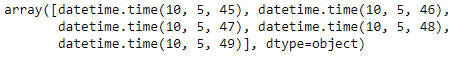Python | Pandas DatetimeIndex.time

• Last Updated : 24 Dec, 2018

Python is a great language for doing data analysis, primarily because of the fantastic ecosystem of data-centric python packages. Pandas is one of those packages and makes importing and analyzing data much easier.

Pandas DatetimeIndex.time attribute outputs an Index object containing the time values present in each of the entries of the DatetimeIndex object.

Syntax: DatetimeIndex.time

Return: numpy array of python datetime.time

Example #1: Use DatetimeIndex.time attribute to find the time part of the DatetimeIndex object.

 # importing pandas as pdimport pandas as pd  # Create the DatetimeIndex# Here 'H' represents hourly frequencydidx = pd.DatetimeIndex(start ='2000-01-10 06:30', freq ='H',                             periods = 3, tz ='Asia/Calcutta')  # Print the DatetimeIndexprint(didx)

Output :Now we want to find all the time part of DatetimeIndex object.

 # find all the time values present in the objectdidx.time

Output :As we can see in the output, the function has returned an Index object containing the time values present in each entry of the DatetimeIndex object.

Example #2: Use DatetimeIndex.time attribute to find the time part of the DatetimeIndex object.

 # importing pandas as pdimport pandas as pd  # Create the DatetimeIndex# Here 's' represents secondly frequencydidx = pd.DatetimeIndex(start ='2014-08-01 10:05:45', freq ='S',                              periods = 5, tz ='Asia/Calcutta')  # Print the DatetimeIndexprint(didx)

Output :Now we want to find all the time part of DatetimeIndex object.

 # find all the time values present in the objectdidx.time

Output :As we can see in the output, the function has returned an Index object containing the time values present in each entry of the DatetimeIndex object.

Attention geek! Strengthen your foundations with the Python Programming Foundation Course and learn the basics.

To begin with, your interview preparations Enhance your Data Structures concepts with the Python DS Course. And to begin with your Machine Learning Journey, join the Machine Learning - Basic Level Course

My Personal Notes arrow_drop_up